### Choose language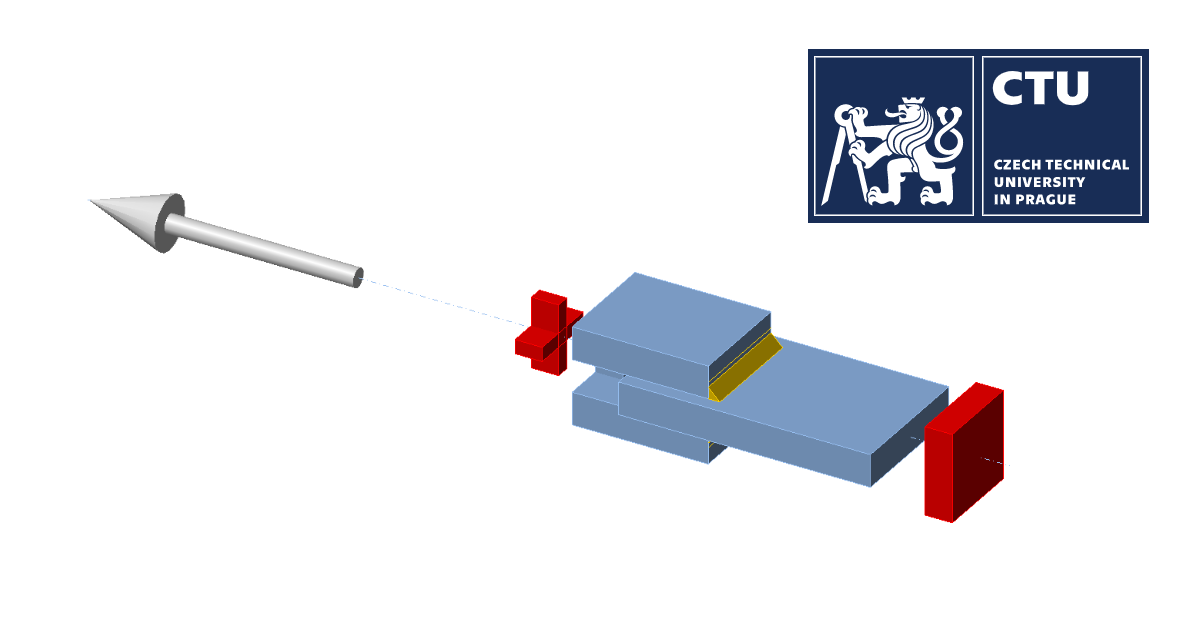# Fire design: Welded lap joint

Component-based finite element method (CBFEM) of a fillet weld in a lap joint during fire is verified with component method (CM).
$$DescriptionThree plates are connected in two configurations, namely with a transverse weld and with a longitudinal weld. The steel grade, length, and throat thickness of the weld are the variable parameters in the study. The joint is loaded by a normal force.The model was created in IDEA StatiCa Connection application.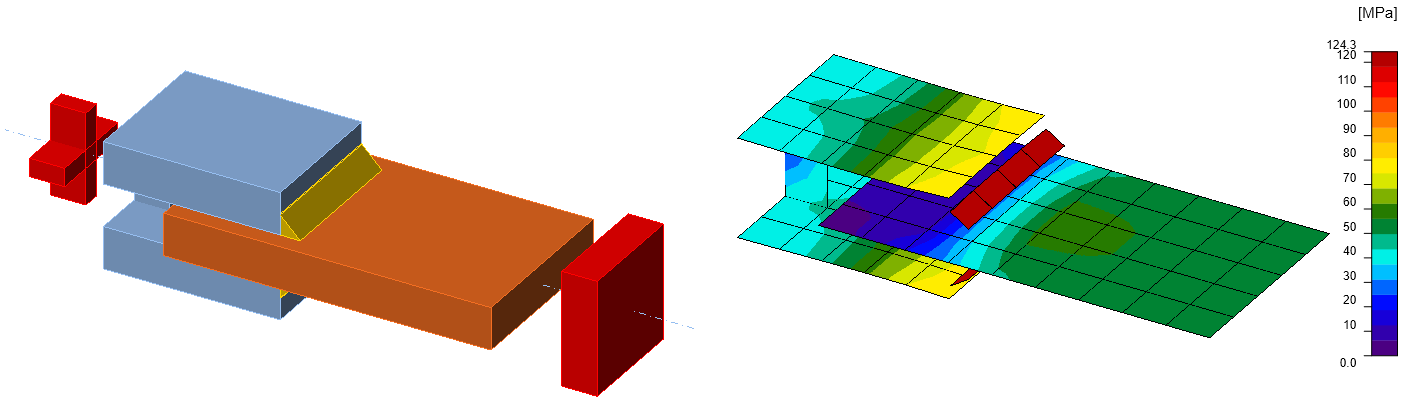Fig. 1: Geometry of welded lap joint with transverse weldAnalytical ModelThe design resistance per unit length of a fillet weld in fire should be determined from:$F_{w,t,Rd}=F_{w,Rd}k_{w,\theta}\frac{\gamma_{M2}}{\gamma_{M,fi}}$where $$k_{w,\theta}$$ is obtained from EN 1993-1-2, Table D.1 for the appropriate weld temperature; $$F_{w,Rd}$$ is determined from EN 1993-1-8, Cl. 4.5.3.VerificationTab. 1: Transverse fillet welds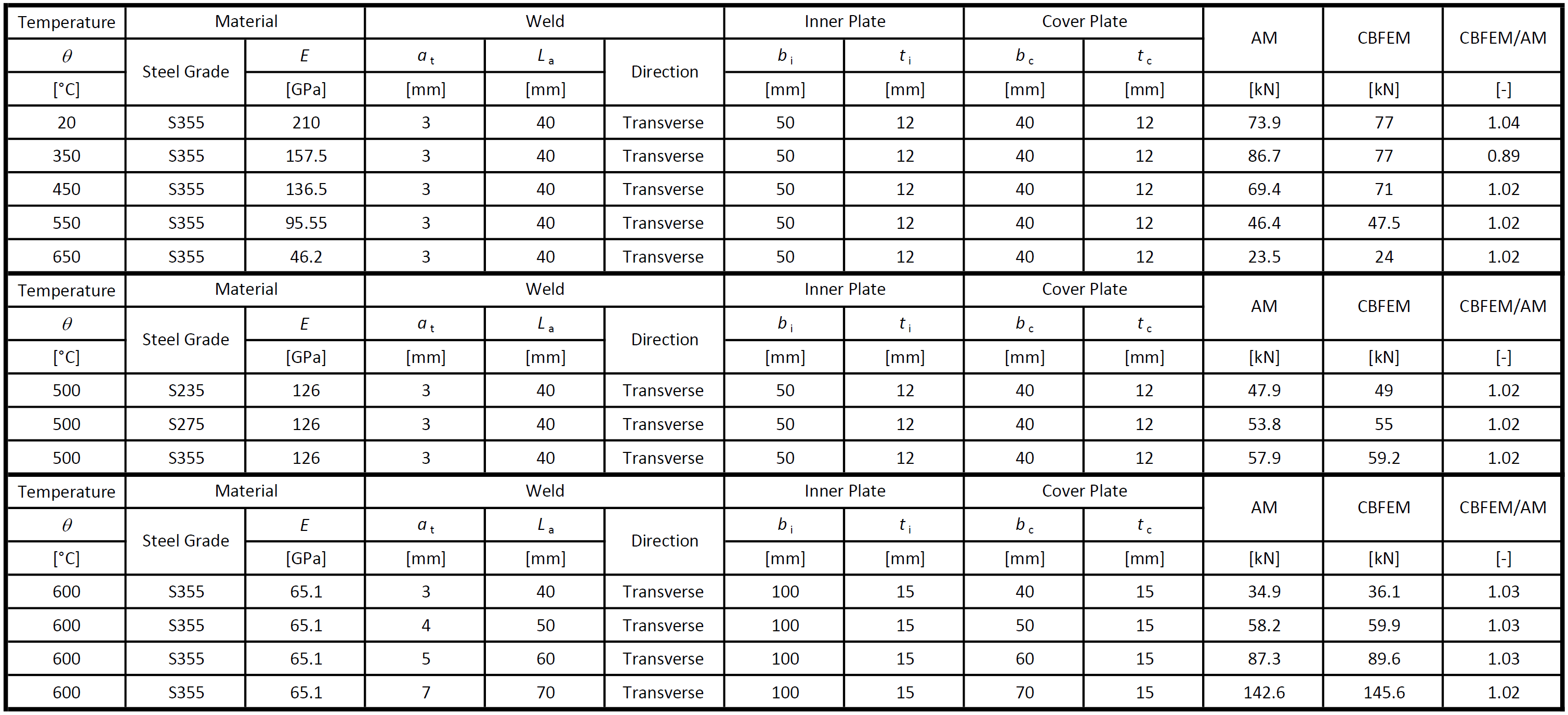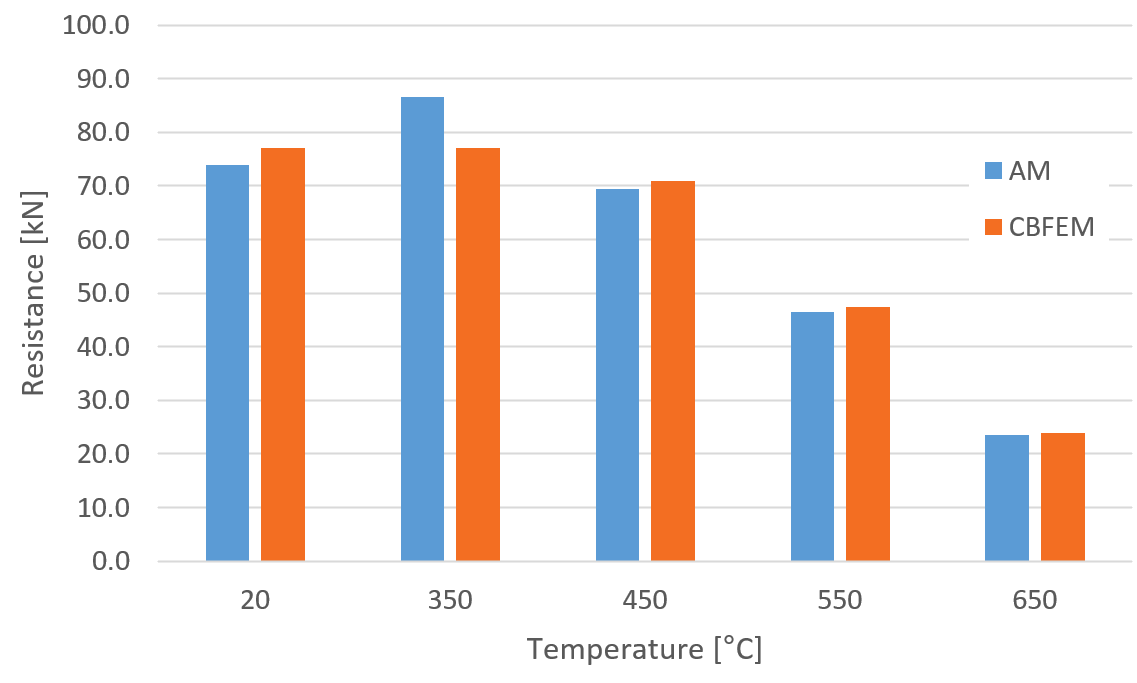Fig. 2: Parametric study of temperature for transverse weld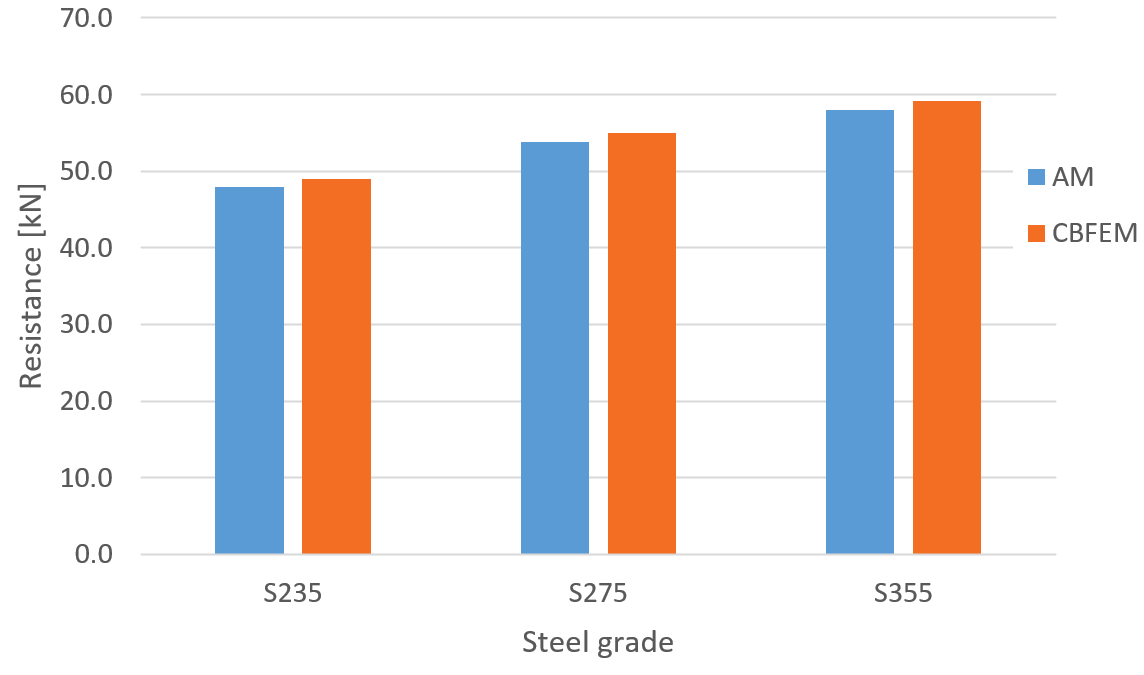Fig. 3: Parametric study of steel grade for transverse weld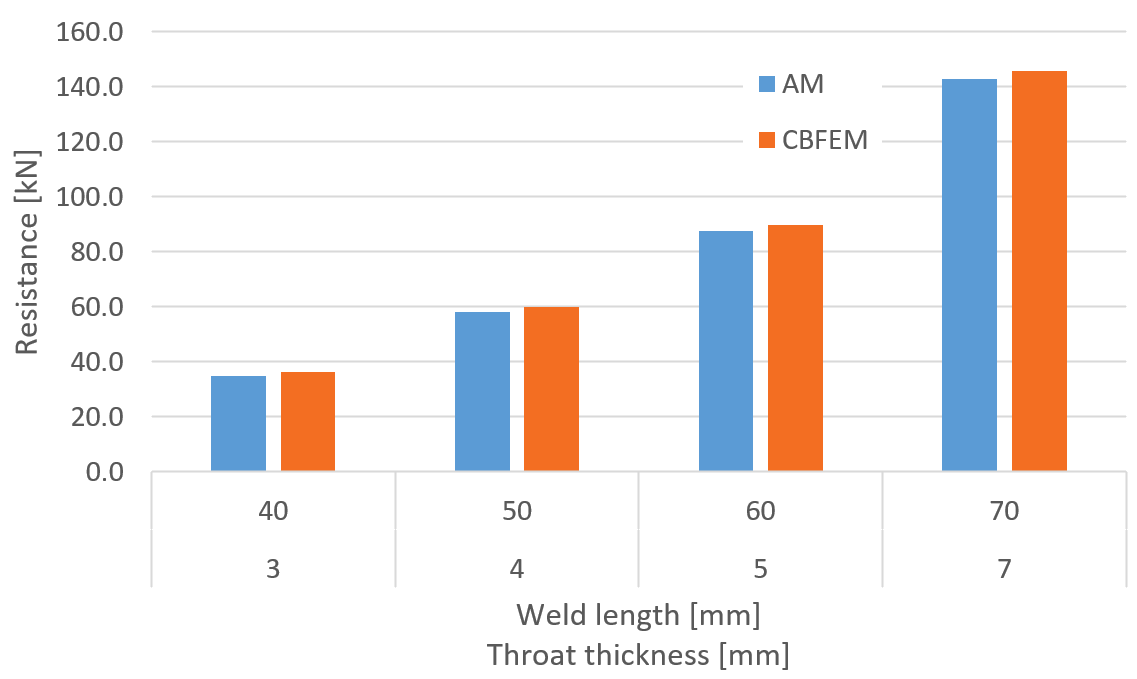Fig. 4: Parametric study of weld length throat thickness for transverse weldThe resistance of transverse fillet welds is in most cases slightly higher (on average by 3 %) in CBFEM than in AM. The exception is the temperature of 350 °C. At this temperature, the resistance of welds is affected only slightly by fire, and the difference between partial safety factors $$\gamma_{M,fi}=1.0$$ and $$\gamma_{M2}=1.25$$ overweight the decrease due to fire. IDEA StatiCa does not allow the bolt or weld resistance to be higher in fire than at room temperature and assumes the minimum of: Load resistance multiplied by reduction factor for temperature, $$k_{\theta}$$, and divided by safety factor for fire design situation, $$\gamma_{M,fi}$$ Load resistance divided by safety factor for bolts and welds, $$\gamma_{M2}$$ Tab. 2: Longitudinal fillet welds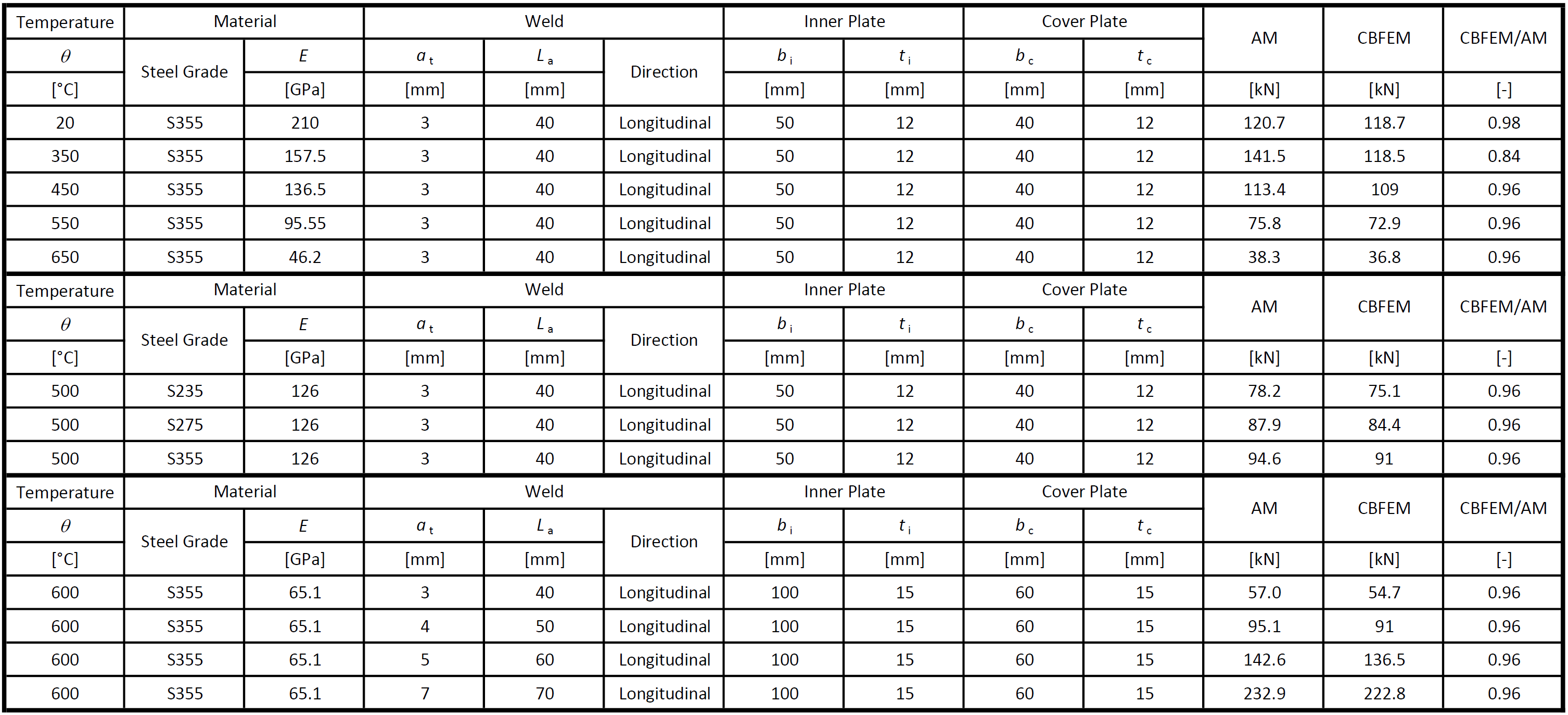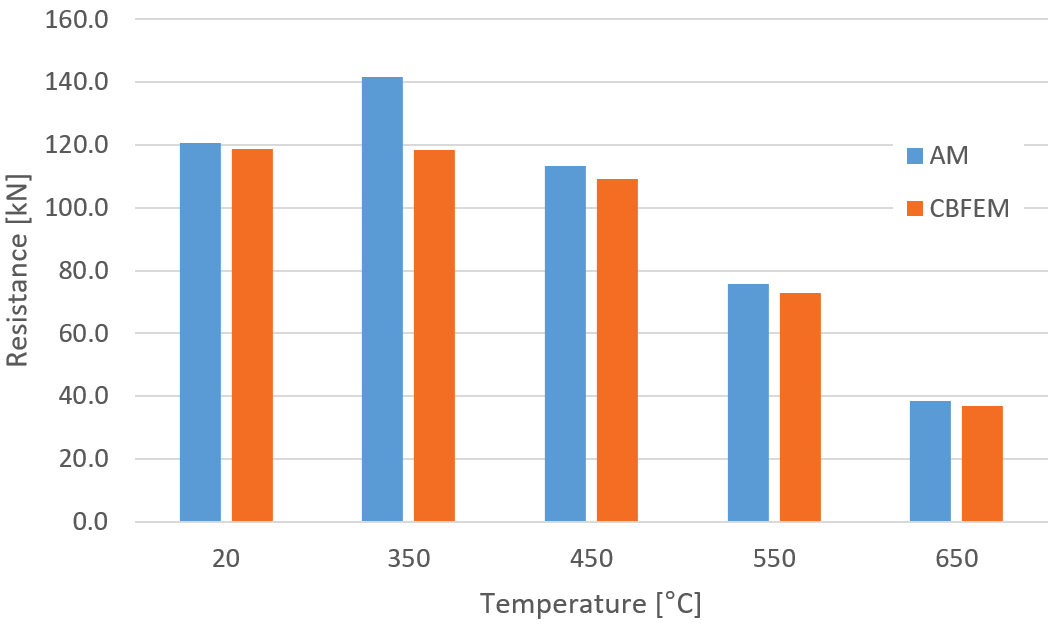Fig. 5: Parametric study of temperature for longitudinal weld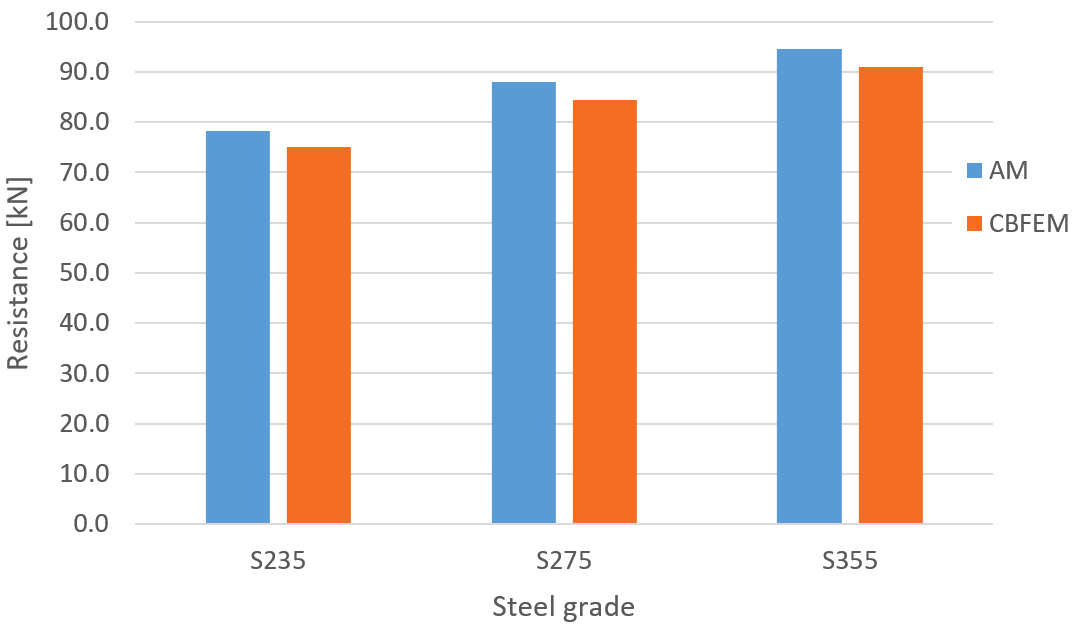Fig. 6: Parametric study of steel grade for longitudinal weld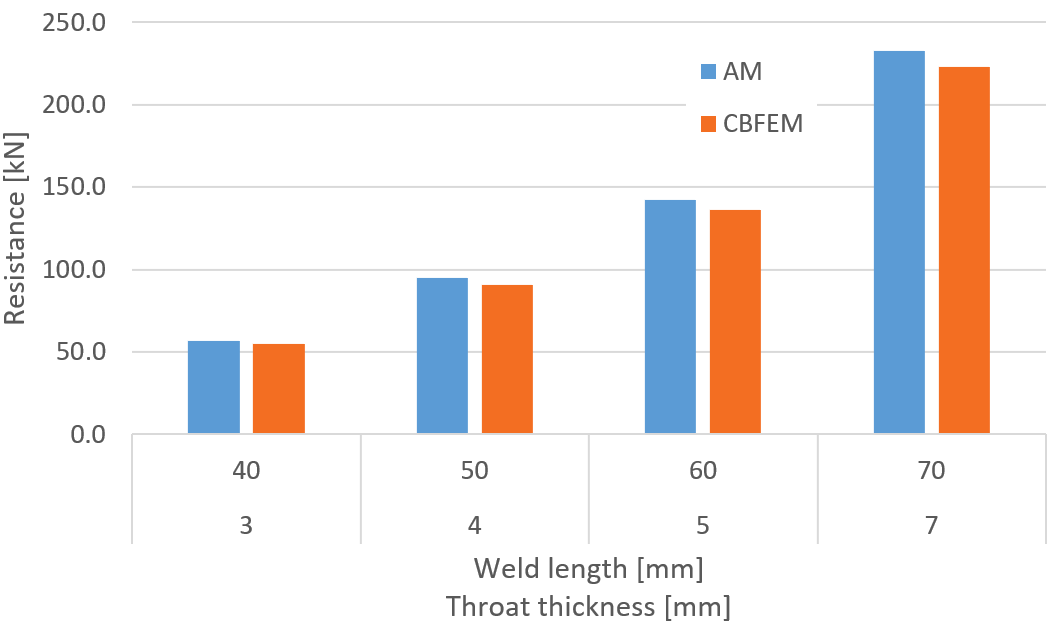Fig. 7: Parametric study of weld length throat thickness for longitudinal weldThe resistance of longitudinal fillet welds is in most cases slightly lower (on average by 4 %) in CBFEM than in AM. The exception is the temperature of 350 °C for the same reason as for transverse welds.Benchmark exampleInputsPlate 2-3: Custom I-profile Thickness t2-3 = 12 mm Width b2-3 = 40 mm Steel S355 Plate 1 Thickness t1 = 12 mm Width b1 = 50 mm Steel S355 Model type N-Vy-Vz Weld, transverse fillet welds Throat thickness ab = 3 mm Weld length Lb = 40 mm Temperature: Both members $$\theta=550\,^\circ C$$ Output Design resistance in tension FRd = 48 kN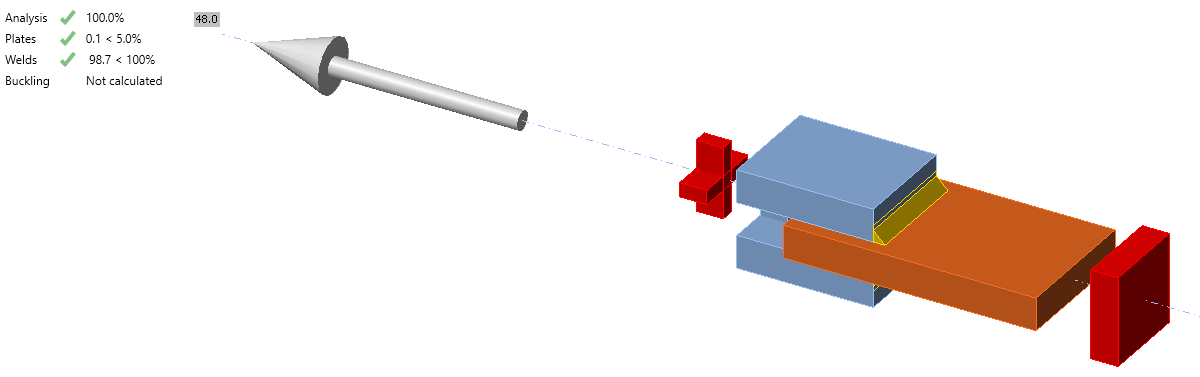Fig. 8: Benchmark example for the welded lap joint Sample files Open in Viewer Download For other verification studies, visit our Support Center - Verifications.$$